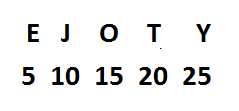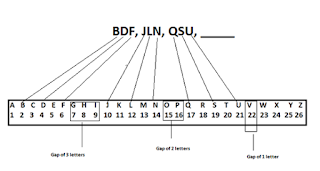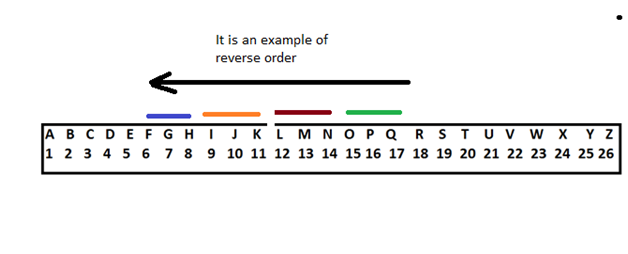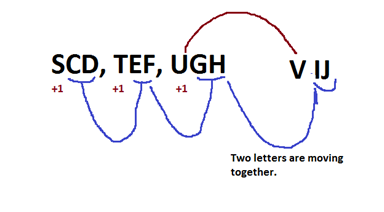New Students Offer - Use Code HELLO

# Alphabet Series Tricks - PDF

Before beginning the Alphabet Series ,let me ask a question

Suppose you are asked find the missing term in the following series

1, 3, 5, 7, __

You can tell immediately that the answer is 9.

Similar thing can be asked in Alphabet Series like:
A, C, E, G, __

Your answer would be ‘I’ because you understood it quickly that  like the number series you need to skip one letter and reach the next letter.

So there are two basic methods to do the Alphabet Series.

1. Remember all the alphabets and their place number , You can even note down during your exam.Here you should remember two things

A to M =  1  to  13    and  N to  Z   =   14  to 26

Intervals like:So if you remember these intervals,It would be helpful in solving alphabet series problems.For example:

### What is the value of U?

So here in this case you can tell immediately that it is 21 because you know that immediately before U is T and its value is 20

Let us pick up another question

### What is the missing letter in the series? U O I ___ A.

Solution: If you observe it attentively, then we can see that they are the vowels written in the REVERSE order.

U   O    I    E   A

Ans. E

### Find the missing term in the series - AD , GJ, MP, ?

Solution:
To do this we need to see their numeral values

A D  =  1 and 1+3 = 1 4

G J  =4+3  is 7 and 7+3 is 10 = 7 10

MP = 10+3 is 13 and 13+3 is 16 = 13 16

So the next would be 16+3 is 19 which means S and 19+3 is 22 which means V = SV

The missing term is SV.

2.Next method is by using circle of letters

This method is very useful when the series is not linear like the one you did in the first method.

Let us understand it through an example:

### BDF, JLN, QSU, ____

Solution:As you can see that between the GROUPS , the gap is reduced by one letter in every step but letters of the group have a gap of one letter only.

BDF, JLN, QSU, WYA

NowAfter identifying the first letter of the group which is W if you look at the circle it is quite easy to reach on the next two letters where as in case of linear series you have to come again which might bring chances of errors.

### Find the next term of the series - AZ, CX, FU, __There two series in this question running simultaneously.### What would be the next term in the following series - QPO, NML, KJI, ____.

Solution:  The next term is HGF.### What is the next term in the series - JAK, KBL, LCM, MDN, ____.

Solution: Each letter in triplets  is  moving one place forward.

Remember: Do not always think that there is a relation between the letters of the group, rather you can you can get a situation where each letter in the group is moving forward or backward to make the next term of the series.

### Find the next term in the series - SCD, TEF, UGH, ____?

Solution: